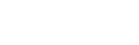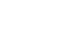# How to Implement the Logistic Sigmoid Function in Python

In this tutorial, I’ll show you how to implement a logistic sigmoid function in Python.

I’ll explain what the logistic sigmoid function is.

I’ll show you how to define the syntax for the logistic sigmoid function in Python.

And I’ll show you a few examples of how it works.

If you need something specific, you can click on any of the following links.

## Introduction to the Logistic Sigmoid Function

The logistic sigmoid function is an s-shaped function that’s defined as:

(1)When we plot it, it looks like this:This sigmoid function is often used in machine learning. In particular, it’s often used as an activation function in deep learning and artificial neural networks.

Since it’s often used in machine learning and deep learning, it’s potentially useful to know how to implement it in common machine learning programming languages.

That being the case, let’s look at how we can implement the function in Python, one of the most popular programming languages for machine learning.

## The syntax for a Python logistic sigmoid function

We can define the logistic sigmoid function in Python as follows:(You can also find the Python code in example 1.)

Here, the def keyword indicates that we’re defining a new Python function.

We’ve named the function “logistic_sigmoid” (although we could name it something else).

The input value is called x.

In the body of the function, we see a return statement and a computation inside of it. This computation is calculating the value:

(2)… where x is the input value to the function.

Notice that to perform this computation, we’re calling the Numpy exponential function.

#### Format of the input values

Let’s talk about the possible input values of the function.

This function will operate on:

• single numbers
• Numpy arrays
• array-like objects (such as Python lists)

#### The output of the function

The output will vary slightly, depending on the input type.

If the input is a number, then the output will be a number.

If the input is an array or array-like object, then the function will output a Numpy array. Importantly, the output array will have the same size and shape as the input. The values of the output array will be the element-wise computation of the input values.## Examples: how to implement and use a logistic sigmoid function in Python

Now that we’ve looked at the syntax for how to implement the logistic sigmoid function, let’s actually execute the function code and use it on some examples.

Examples:

### Preliminary code: Import Numpy and Set Up Plotly

Before you run the examples, you’ll need to run some setup code.

Specifically, you need to import some packages, and set up Plotly to render images.

#### Import Packages

First, we need to import Numpy and Plotly express.

import numpy as np
import plotly.express as px


We’re going to use a Numpy function – np.exp – in our implementation of the function.

And we’ll use Plotly Express to plot the function in example 6.

##### Set Up Image Rendering

Next, you might need to configure Plotly to render images on your system.

To be clear: you only need to do this if you’re using an IDE.

By default, Plotly is set up to render images (i.e., output visualizations) in a browser window. That’s fine if you’re working in a notebook. But if you’re working in an IDE like PyCharm or Spyder, it will cause errors.

So, you need to tell Plotly to render its output as an svg directly in the IDE.

(Note: if you’re using Jupyter, you can skip this code!)

import plotly.io as pio
pio.renderers.default = 'svg'


After you’ve run the setup code, you should be ready to run these examples.

### EXAMPLE 1: Define the Logistic Sigmoid Function using Python

First, we’ll define the logistic sigmoid function in Python:

def logistic_sigmoid(x):
return(1/(1 + np.exp(-x)))


#### Explanation

Here, we’re using Python’s def keyword to define a new function. We’ve named the new function “logistic_sigmoid”.

The function has one input: x.

The function will return the following:

(3)Notice that we’re computing this output in part by using the Numpy exponential function.

### EXAMPLE 2: Compute the logistic sigmoid of 0

Now that we have our function defined, let’s compute the sigmoid of 0

logistic_sigmoid(0)


OUT:

0.5


### EXAMPLE 3: Compute logistic sigmoid of 5

Next, let’s compute the sigmoid of 5.

logistic_sigmoid(5)


OUT:

0.9933071490757153


#### Explanation

Here, we’ve computed the logistic sigmoid of 5.

Notice that the value is very close to 1.

This is expected. As x goes to infinity, the logistic sigmoid function will converge to 1.

### EXAMPLE 4: Compute logistic sigmoid of -5

Now, let’s compute the sigmoid of -5.

logistic_sigmoid(-5)


OUT:

0.0066928509242848554


#### Explanation

Here, we’ve computed the sigmoid of -5.

Notice that the value is very close to 0.

This is as we would expect. As x goes to negative infinity, the function will converge to 0.

### EXAMPLE 5: Use the logistic sigmoid function on an array of numbers

Now, we’ll use our sigmoid function on an array of numbers.

#### Create Numpy Array

First, we’ll create a Numpy array.

In particular, we’re going to create an array of evenly spaced values.

To do this, we’ll use Numpy linespace function, and create an array of evenly spaced values from -10 to 10.

x_values = np.linspace(start = -10, stop = 10, num = 201)


Let’s print it out to take a look:

print(x_values)


OUT:

[-10.   -9.9  -9.8  -9.7  -9.6  -9.5  -9.4  -9.3  -9.2  -9.1  -9.   -8.9
-8.8  -8.7  -8.6  -8.5  -8.4  -8.3  -8.2  -8.1  -8.   -7.9  -7.8  -7.7
-7.6  -7.5  -7.4  -7.3  -7.2  -7.1  -7.   -6.9  -6.8  -6.7  -6.6  -6.5
-6.4  -6.3  -6.2  -6.1  -6.   -5.9  -5.8  -5.7  -5.6  -5.5  -5.4  -5.3
....
5.6   5.7   5.8   5.9   6.    6.1   6.2   6.3   6.4   6.5   6.6   6.7
6.8   6.9   7.    7.1   7.2   7.3   7.4   7.5   7.6   7.7   7.8   7.9
8.    8.1   8.2   8.3   8.4   8.5   8.6   8.7   8.8   8.9   9.    9.1
9.2   9.3   9.4   9.5   9.6   9.7   9.8   9.9  10. ]


I’ve abbreviated the output somewhat for space.

But if you look closely, you can see, x_values contains the values from -10 to 10, in increments of .1.

#### Compute Logistic Sigmoid Values

Now, we’re going to use our sigmoid function on x_values.

logistic_sigmoid_values = logistic_sigmoid(x_values)


And let’s print the results:

print(logistic_sigmoid_values)


OUT:

[4.53978687e-05 5.01721647e-05 5.54485247e-05 6.12797396e-05
6.77241496e-05 7.48462275e-05 8.27172229e-05 9.14158739e-05
1.01029194e-04 1.11653341e-04 1.23394576e-04 1.36370327e-04
1.50710358e-04 1.66558065e-04 1.84071905e-04 2.03426978e-04
....
9.99849290e-01 9.99863630e-01 9.99876605e-01 9.99888347e-01
9.99898971e-01 9.99908584e-01 9.99917283e-01 9.99925154e-01
9.99932276e-01 9.99938720e-01 9.99944551e-01 9.99949828e-01
9.99954602e-01]


#### Explanation

I’ve abbreviated the results, but here, we can see roughly what’s in the results.

If you look closely, you can see some values that are very close to 0 and also some values that are very close to 1.

(We’ll see all of this in a moment when we plot the data.)

### EXAMPLE 6: Plot the Logistic Sigmoid function

Finally, let’s plot the logistic sigmoid function.

Specifically, we’ll plot the output values we just computed in example 5.

To do this, we’ll use the Plotly line function, px.line.

px.line(x = x_values, y = logistic_sigmoid_values)


OUT:#### Explanation

Here, we plotted the logistic sigmoid values that we computed in example 5, using the Plotly line function.

On the x-axis, we mapped the values contained in x_values.

On the y-axis, we mapped the values contained in the Numpy array,  logistic_sigmoid_values.

The resulting output is a plot of our s-shaped sigmoid function.

Do you have other questions about how to create or use a logistic sigmoid function in Python?

In this tutorial, I’ve explained how implement and use a logistic sigmoid in Python, using Numpy.

If you’re serious about mastering Numpy, and serious about data science in Python, you should consider joining our premium course called Numpy Mastery.

Numpy Mastery will teach you everything you need to know about Numpy, including:

• How to create Numpy arrays
• How to reshape, split, and combine your Numpy arrays
• What the “Numpy random seed” function does
• How to use the Numpy random functions
• How to perform mathematical operations on Numpy arrays
• and more …

Moreover, this course will show you a practice system that will help you master the syntax within a few weeks. We’ll show you a practice system that will enable you to memorize all of the Numpy syntax you learn. If you have trouble remembering Numpy syntax, this is the course you’ve been looking for.

Find out more here:

Joshua Ebner

Joshua Ebner is the founder, CEO, and Chief Data Scientist of Sharp Sight. Prior to founding the company, Josh worked as a Data Scientist at Apple. He has a degree in Physics from Cornell University.

### 3 thoughts on “How to Implement the Logistic Sigmoid Function in Python”

1. How do you just the size of the curves?